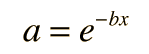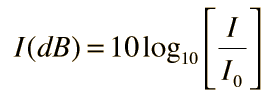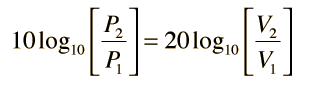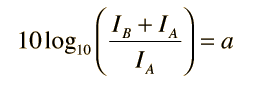# Exponentials and Natural Logs

Sometimes the quantity which is to be solved for in an equation is in an exponential functionThe solution can be found by taking the natural logarithm of both sides of the equation.so thatApplications of logs Applications of exponentials
Index

 HyperPhysics*****HyperMath*****Logarithms R Nave
Go Back

# Applications of Logarithms

Applications of common logarithms include

The sound intensity in decibels:The relative electric power in dB:Decibels of ratios in the treatment of masking :Index

 HyperPhysics*****HyperMath*****Logarithms R Nave
Go Back

# Applications of Exponentials

Applications of exponentials include

The charging of a capacitor :Index

Exponents

 HyperPhysics*****HyperMath*****Logarithms R Nave
Go Back